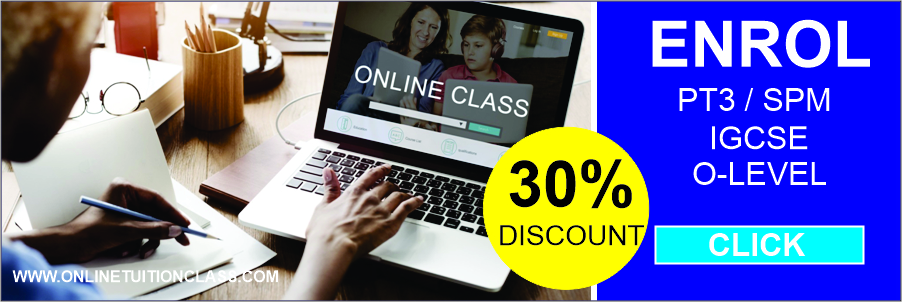### Add Math IGCSE Online Class

•by yawhuat
• Jan 30, 2023
• 0
• Category: ,

# ONLINE ADD MATH CLASS FOR IGCSE STUDENTS

Cambridge IGCSE Additional Mathematics encourages STUDENTS to grasp and further expand their knowledge of Mathematics. Students enrolling for Add Math are usually math enthusiasts. Most students were asking about the relevancy of Add Math and we would like students to enroll in this subject if they are planning to venture into engineering courses in the future. Add Math is not really difficult but it does pack with more algebras. Among the key topics to Add Math are:-

• Equations, inequalities, and graphs
• Indices and surds
• Factors of polynomials & simultaneous equations
• Logarithmic and exponential functions
• Straight-line graphs & circular measure
• Trigonometry
• Permutations and combinations
• Series & Vectors in two dimensions
• Differentiation and integration
• Probability and Statistics
• Geometry
• Permutation and Combination
• Solutions of Triangles